# Heat Transfer Enhancement in Subchannel Geometry of Pressurized Water Reactor Using Water-Based Yttrium Oxide Nanofluid

Heat Transfer Enhancement in Subchannel Geometry of Pressurized Water Reactor Using Water-Based Yttrium Oxide Nanofluid

Petroleum Engineering Department, University of Kerbala, Karbala 56001, Iraq

Corresponding Author Email:
farhan.lefta@uokerbala.edu.iq
Page:
979-986
|
DOI:
https://doi.org/10.18280/ijht.390335
23 April 2020
|
Accepted:
17 March 2021
|
Published:
30 June 2021
| Citation

OPEN ACCESS

Abstract:

Simulation of Computational Fluid Dynamic is applied to present the thermal performance of water-based Yttrium oxide nanofluid in subchannel of pressurized water reactor (PWR) system. Thermal hydraulic aspect such as pressure drop and heat transfer are estimated in typical conditions of pressurized water with flow rates ranged (20×103≤Re≤80×103) using fresh water (0 %vol.) and different volume fraction of water-Yttrium oxide nanofluid (2 and 4% vol.) as coolant fluid. Results were obtained and compared with correlations of single-phase pressure drop and convective heat transfer for the case of fully developed turbulent flow. The addition of Yttrium oxide nanoparticles to the coolant fluid in pressurized water reactor led to increase in convective heat transfer coefficient and pressure drop. Increasing the nanoparticle volume fraction of (2 and 4% vol.) causing an increase in the average Nu by 3.46% and 7.61%, respectively. The CFD model established in ANSYS software was validated by comparing the pressure drop of CFD results with Blasius correlation and Nu with Ma¨ıga et al. correlation and gave a good agreement.

Keywords:

ANSYS fluent, CFD, PWR, subchannel geometry, water-based nanofluids

1. Introduction

The thermal performance of coolant fluid used for cooling the fuel rod bundles (shown in Figure 1) of the pressurized water reactor is very important for the design and safe operation viewpoint. In such type of nuclear reactor, controlling of pressure and fluid temperature are of the priority for safety operation. For instance, a spot with high temperature in the cladding of fuel rod is considered as danger case. The most common nuclear reactors that use water as coolant fluid are the pressurized water reactor. The boiling point of the coolant fluid in these reactors is high due to the high-water pressure, and the fluid is always in liquid phase . The important duty of the nuclear power plant is increasing the heat output from the reactor core by increasing the temperature of coolant fluid in the reactor core outlet. Some types of nanoparticles be with high thermal conductivity which be useful for enhancing heating capacity of the reactor coolant fluid. Base fluids can be replaced by nanofluid which are a good choice for improving the thermal performance of the nuclear power plant .

Previous studies indicate that increasing the nanoparticles concentration in the base fluid enhances both the thermal conductivity and heat transfer [2, 3]. Using nanofluid as coolant in the nuclear reactor will rises the critical heat flux, which leads to enhance the removal from nucleate boiling ratio of the coolant fluid [4-6].

Conner et al.  used a computational fluid dynamic scheme to simulate single-phase fluid with steady state conditions in the pressurized water reactor fuel rod assembly and achieved tests to validate the results. The numerical results were compared with the experimental and found that the computational fluid dynamic model is appropriate for estimating the behavior of coolant fluid in PWR fuel rod assembly. Kamal and Zahra  used silver oxide nanofluid as enhancer of a twin heat transfer and controlling the surplus reactivity in VVER-1000 which be a type of pressurized water. The coolant fluid in the first loop of this type of reactor is replaced by nanofluid to act as neutron moderator, neutron absorber, and coolant fluid. The volume fraction of 2% is found to suitable for both thermohydraulics and neutronics safety of the VVER-1000 nuclear power reactor. Abdellahoum et al.  performed CFD analysis for turbulent forced convection of water-based (Cu, CuO, Ag and Al2O3) nanofluid over heated cavity in horizontal duct test section. The analysis is carried out using ANSYS software ver. 14.0 code. The increase in volume fraction of nanoparticles increases the average Nusselt number for the tested range of Reynolds number. Moghadassiet et al.  achieved computational fluid dynamic modeling on horizontal tube of circular cross section to study the effect of volume fraction of nanoparticles on heat transfer of laminar forced convection. They used water-based Al2O3 and Al2O3-Cu hybrid nanofluid with volume fraction of 0.1%. this hybrid nanofluid gave higher convective heat transfer coefficient. Ghulam et al.  presented a comparative study of flow velocity, pressure, moderator temperature, fuel temperature at the fuel pin cell, and moderator density of pressurized water reactor. The thermal performance is depended on mass and enthalpy equation of the coolant/moderator with one group diffusion equation of the fuel pin. Sierchula  studied in separate the passive containment cooling system and passive residual heat removal system for the nuclear power plant. The outcome of this study is simulating, presenting and describing some of the most important parameters in the nuclear power plant such as reactor coolant temperature and heat transfer. Bikash Poudel et al.  performed simplified dynamic model of integral pressurized water reactor type, to estimate the supporting of the nuclear reactor to the dynamics of power plant system. This model is engaged with the modified turbine system and a resulted power system is simulated according to the processes of heat generation and heat transfer based on point kinetic and primary coolant. The responses of power system and internal reactor are when applying electrical demand of 20% electrical output with 80% of valve opening rate.

This paper presents the heat transfer enhancement in the suchannel geometry of the pressurized water reactor system using water-based Yttrium oxide nanofluid and validate the CFD results with the correlations available in the literature.

## 1.pngFigure 1. Front and top view of fuel rod assembly

2. Methodology

2.1 Theoretical modelling

The coolant water in the pressurized water reactor flowing in parallel to the fuel rod bundle, the unit channel is known as subchannel. Modelling this subchannel was made extensively before using the flow symmetry modelling [14-18]. FLUENT 16.0 is used to solve the steady-state Reynolds-Averaged Navier-Stokes, energy, mass, and turbulent equations. The continuity, momentum, and energy equations are listed in Eqns. (1-3) :

$\frac{\partial \rho u_{i}}{\partial x_{i}}=0$     (1)

\begin{aligned} \frac{\partial u_{i} u_{j}}{\partial x_{j}}=-\frac{\partial P}{\partial x_{i}}+& \frac{\partial}{\partial x_{j}}\left[\mu\left(\frac{\partial u_{i}}{\partial x_{j}}+\frac{\partial u_{j}}{\partial x_{i}}\right)-\rho\left(u_{i}^{\prime} u_{j}^{\prime}\right)\right] \\ &+\rho g_{i} \end{aligned}     (2)

$\frac{\partial}{\partial x_{i}}\left(u_{i}(\rho E+P)\right)=\frac{\partial}{\partial x_{i}}\left(K_{e f f} \frac{\partial T}{\partial x_{i}}+u_{j}\left(\tau_{i j}\right)_{e f f}\right)$     (3)

2.2 Boundary conditions

The coolant water enters the subchannel with uniform temperature of 293 K and different velocity values. The velocity of the inlet coolant water was applied with different values, which are listed in Table 1). The available correlations were chosen to validate the CFD model Reynolds number and thermal boundary conditions . A relative average pressure equaling zero was defined the outlet of the computational model. The surfaces of fuel rod were assumed to be smooth. Applying constant heat flux of 584 KW/m2 on the outer fuel rod surface. Bianco et al. formula [15, 20-22] based on thermophysical properties of the nanofluid with different volume fractions of 2 and 4% vol. at room temperature has been chosen to estimate the Reynolds number (Re = (ρ·v·Dh)/μ). Assumption of this work can be summarized as: steady state condition, constant heat flux, incompressible fluid, Newtonian fluid, and turbulent flow.

Table 1. Inlet velocities (m/s) and Reynolds numbers

 Re $\varphi$=0 $\varphi$=2% $\varphi$=4% 20x103 6.57 7.26 8.43 40x103 13.13 14.52 16.86 60x103 19.70 21.75 25.28 80x103 26.26 29.04 33.71

2.3 Thermo-physical properties of nanofluids

In practical applications, nanofluids contain the oxide particles with finer size up to 40 nm . In the present work the used nanofluids is water contained nanoparticles of Y2O3 with mean particle size of 40 nm. In order to estimate the density, heat capacity, dynamic viscosity, and thermal conductivity of water-based nanofluids, it will be needed to use the following equations [14, 20-24],

$\rho_{n f}=(1-\varphi) \rho_{b f}+\varphi \rho_{p}$      (4)

$\left(c_{p}\right)_{n f}=(1-\varphi)\left(c_{p}\right)_{b f}+\varphi\left(c_{p}\right)_{p}$      (5)

$\mu_{n f}=\left(123 \varphi^{2}+7.3 \varphi+1\right) \mu_{b f}$      (6)

$k_{n f}=\left(4.97 \varphi^{2}+2.72 \varphi+1\right) K_{b f}$      (7)

The thermophysical properties of water-based nanofluid and Y2O3 nanoparticle are listed in Tables 2 and 3, respectively.

Table 2. Thermophysical properties of nanofluid with different nanoparticle volume fractions at 293 K

 $\varphi$ (%) $\rho$(kg/m3) $C_{p}$(J/kg.K) k (W/m. K) $\mu$(Pa. s) 0 1000 4182 0.597 0.000998 2 1080 4107 12.677 0.001192 4 1160 4032 13.401 0.001486

Table 3. Y2O3 nanoparticle at 293 K

 $\rho$(kg/m3) $C_{p}$ (J/kg.K) k (W/m. K) 5000 440 12

2.4 Grid independency study

The influence of mesh size on the sensitivity of results must be studied for the first step of accomplishing a CFD simulation. For more accurate approach, a more nodes are needed, and using further nodes will escalates the requisite computational time and computer memory. In order to determining the suitable number of nodes, it can be done by increasing the nodes number until the mesh being fine. Figure 2 presents the temperature variation with grid cell number for 4% vol. and Re=80×103. It was shown that the increase in cell number increases temperature until reaching to approximately constant value.

## 2.png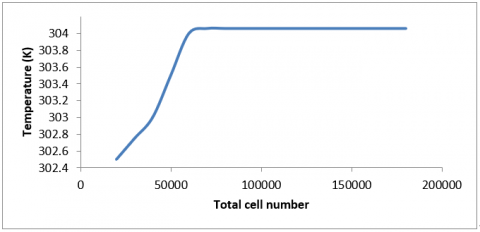Figure 2. Grid independency study for temperature of 4% vol. and Re=80×103

2.5 Convergence

A solution was converged when all conservation differential equations (continuity equation, X-velocity, Y-velocity, Z-velocity, energy equation, and $k-\varepsilon$) were subjected to a specified tolerance at all points. Therefore, the monitoring the convergence of the solution was very important. Figure 3 shows convergence of volumetric flow rate at the outlet. It can be seen that the convergence occur at 200 iterations.

## 3.png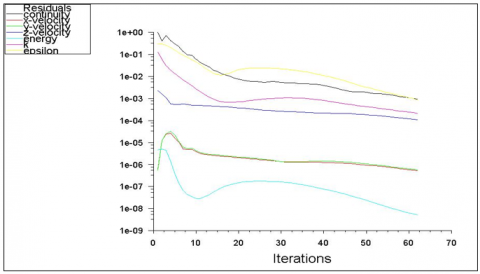Figure 3. Convergence of iterations

3. Results and Discussion

The CFD results are obtained and evaluated in terms of average heat transfer coefficient, Nusselt number, and pressure drop for the inlet coolant flow velocity corresponding to Reynolds number of 20×103, 40×103, 60×103, and 80×103, with Y2O3 volume fraction of 0, 2, and 4%. The single-phase approach with constant heat flux of 584 W/m2 is used to obtain the results. In all cases the particles size was considered equal to 40 nm.

The validation of the water-based Y2O3 nanofluids has been achieved using the correlations in the literature and considering a fully developed turbulent flow as a function of average Nusselt number and pressure losses for smooth wall pipe. The widely used correlation suggested by Maïga et al.  (8) is used to validate the average Nusselt number:

$N u=0.085 R e^{0.71} P r^{0.35}$                (8)

$10^{4} \leq R e \leq 5 x 10^{5}$

$6.6 \leq P r \leq 13.9$

$0 \leq \varphi \leq 10 \%$

Correlation of Eq. (8) is used to calculate the heat transfer coefficient for the water-based Y2O3 nanofluid flowing in smooth tube under the condition of constant heat flux.

3.1 Velocity profile

The velocity profile along the center of coolant fluid passing through the suchannel of 5% vol. is presented in Figure 4. It is noted that, the non-dimensional centerline velocity (V/Vin) increases as Reynolds number decrease, and decreases with non-dimensional flowing distance (Z/L).

## 4.png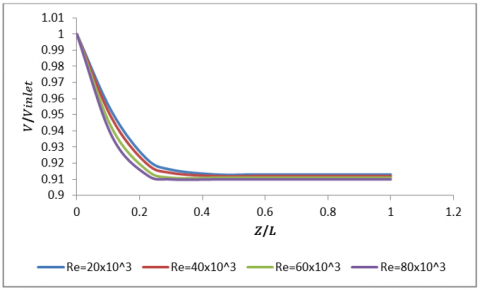Figure 4. Velocity distribution at different locations for 4% vol. nanofluid

## 5.png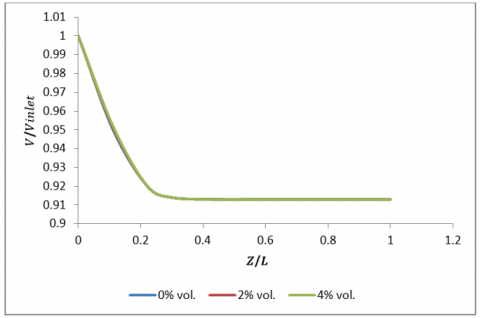Figure 5. Velocity distribution for different % vol. and Re=80×103

## 6.png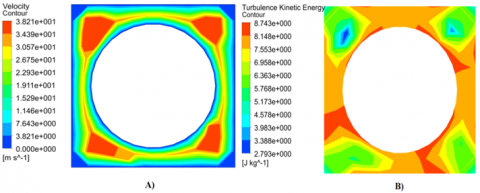Figure 6. A) local velocity and B) turbulent kinetic energy at the coolant water outlet for Re=80×103 and 4% vol

The effect of varying the volume fraction of nanoparticles ( $\varphi$= 0%, 1% and 4% vol.) on the velocity distribution along the centerline of water-based Y2O3 nanofluid with constant Reynolds number (Re= 80×103) is presented in Figure 5. It is clear that the increase in volume fraction of Y2O3 nanoparticles does not affect the velocity magnitude in accordance with [15, 19]. The velocity and turbulent kinetic energy contours at the subchannel outlet for nanoparticle volume fraction of 4% and Re=80×103 are presented in Figure 6.

3.2 Temperature profile

Contours of temperature profile at the subchannel outlet for different particle volume fractions (0, 2, and 4% vol.) are shown in Figure 7 A, B, and C, respectively. It is clear that the presence of nanoparticles in coolant fluid has an advantageous effect on the bulk and wall temperatures compared to the case of fresh coolant water.

The temperature profile along the coolant fluid centerline with different nanoparticle volume fraction is shown in Figure 8. It is clear that temperature profile of coolant water increases for all values of nanoparticle volume fractions. The coolant water absorbs heat along moving through the subchannel. The bulk temperature of fresh water seems to be larger than the case of 2% and 4% volume fraction. The presence of nanoparticles make constant heat flux along the fuel rod wall for all value of volume fractions, and thus lead to decrease the temperature profile with increasing nanoparticle volume fraction.

## 7.png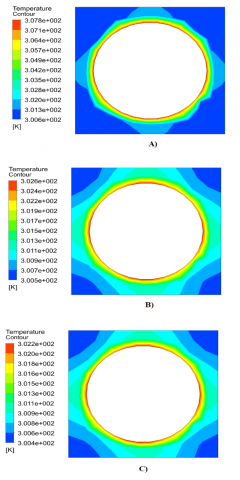Figure 7. Contour of local temperature at the channel outlet for Re=80×103, A) 0%, B) 2%, and C) 4% vol

## 8.png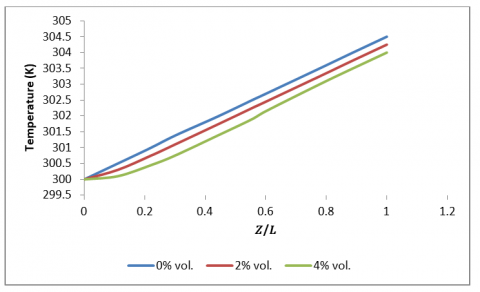Figure 8. Temperature distribution along the channel centerline and Re=80×103

3.3 Pressure drop

The efficiency of nanofluid application can be determined by the coolant fluid pressure drop in heated subchannel. The coolant fluid pumping power and pressure drop are carefully concerned. The pressure profile in the coolant fluid with different flow Reynolds number and nanoparticle volume fraction is described in Figure 9. The pressure drop seems to be increased with increasing Reynolds number for all volume fractions and that the pressure drop of nanofluids increases with the increasing volume fractions as shown in Figure 10. This may attribute to due to the fact that the coolant pressure drop could influenced by the viscosity and density of coolant fluid.

The Moody chart or other available correlation can be used to determine the friction factor of the subchannel geometry. The friction factor of single-phase turbulent flow Reynolds number 2300<Re<100×103 for smooth pipe can be calculated by the Blasius correlation:

$f=\frac{0.3164}{R e^{0.25}}$     (9)

The drop in pressure caused by friction can be estimated by:

$\Delta P=f\left(\frac{L}{2 D_{h}}\right) \rho v^{2}$      (10)

Eq. (11) can be used to find the effect of nanoparticle in coolant fluid, this achieved by the difference in pressure drop along the coolant fluid in subchannel and compared with base (fresh) fluid.

$d P=\left(\frac{\Delta P_{n f}-\Delta P_{b f}}{\Delta P_{b f}}\right) x 100(\%)$      (11)

Table 4. Difference in pressure drop between 2 and 0%vol

 Re$\Delta P(P a)$ present work (0% vol.) $\Delta P(P a)$ present work (2% vol.) Difference in pressure drop (%) 20x103 1000 1150 15 40x103 6000 7020 17 60x103 16000 18880 18 80x103 26000 30950 19

The result reveals that the Y2O3/water pressure drop make maximum increases by about 19% and 94% for φ=2% and φ=4% of, respectively as shown in Tables 4 and 5.

Table 5. Difference in pressure drop between 4 and 0%vol

 Re $\Delta P(P a)$ present work (0% vol.) $\Delta P(P a)$ present work (4% vol.) Difference in pressure drop (%) 20x103 1000 1900 90 40x103 6000 11520 92 60x103 16000 31040 94 80x103 26000 50440 94

## 9.pngFigure 9. Pressure drop vs. Re for different %vol

## 10.png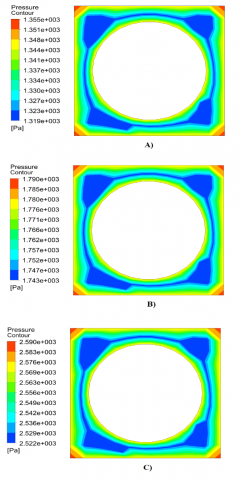Figure 10. Contour of local pressure at the channel outlet for Re=80×103, A) 0%, B) 2%, and C) 4% vol

3.4 Calculations of heat transfer

Heat transfer calculations can be made by applying a constant heat flux to the fuel rod walls along the subchannel and constant temperature at the subchannel inlet for different nanoparticle volume fraction. The local and average heat transfer coefficients are defined as follows:

$h(z)=\frac{Q}{A\left(T(z)_{W}-T(z)_{b}\right)}$      (12)

$q=$ heat flux $=\frac{Q}{A}$, thus

$h(z)=\frac{q}{T(z)_{W}-T(z)_{b}}$       (13)

$h_{a v}=\frac{1}{L} \int_{0}^{L} h(z) d z$       (14)

Calculations of Nusselt number was done by averaging heat transfer coefficient values over the wall region surface, and listed in Table 6. The equation of average Nusselt number is estimated from the following equation:

$N u=\frac{h_{a v} \cdot D}{K_{0}}$     (15)

Figure 11 presents the average heat transfer coefficient for different nanoparticle volume fraction and flow Reynolds number. The heat transfer provided by the inclusion of nanoparticles in comparison with the case of fresh fluid is the useful contribution. The value of heat transfer coefficient is increases with increasing flow Reynolds number and nanoparticle volume fraction. The cause of this increase is from the fact that the improving of thermophysical properties of the nanofluid with respect to the fresh fluid. The fluid with high thermal conductivity of nanoparticles will have higher heat transfer. So, nanoparticles will increase the fluid density, thus it is required to increase the bulk temperature if compared with the case of fresh fluid. Increase the difference in temperature between wall and bulk will excite the increase in the heat transfer coefficient.

## 11.png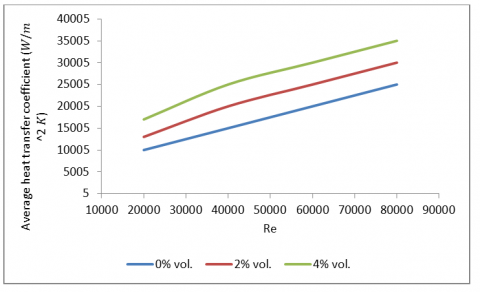Figure 11. Average heat transfer coefficient for different %vol. and Re

Figure 12 shows the variation of the averaged Nusselt number for all volume fraction and Reynolds number. It is clear that average Nusselt number increases with increasing volume fraction by 3.46% for the 2% vol. and 7.61% for 4% vol.

Table 6. Average Nu for different %vol. and Re

 4% Vol. 2% Vol. 0% Vol. Reynolds number 189 174 162 20x103 315 299 287 40x103 440 424 412 60x103 573 549 537 80x103

## 12.png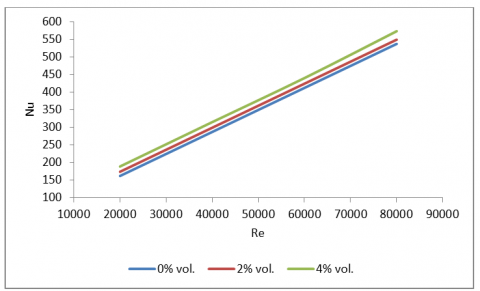Figure 12. Average Nu for different %vol. and Re

3.5 Validation of the CFD results

The CFD Results estimated by ANSYS Fluent were validated by comparing the pressure drop with Blasius correlation and Nu with Maïga et al. . Figures 13, 14, and 15 show the pressure drop-Re results of 0, 2, and 4% vol., respectively with the experimental correlations proposed by Blasius. The CFD results for the pressure drop show a maximum deviation of ±3.58, ±13.42, and ±11.56% for 0, 2, and 4% vol., respectively from the correlation.

## 13.png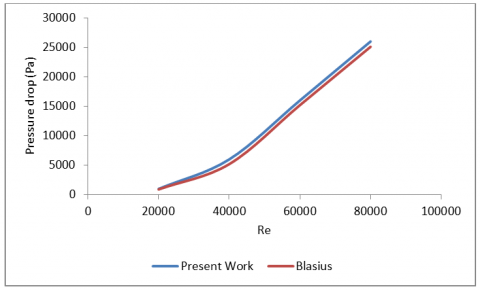Figure 13. Comparison of pressure drop with Blasius correlation for 0% vol

## 14.png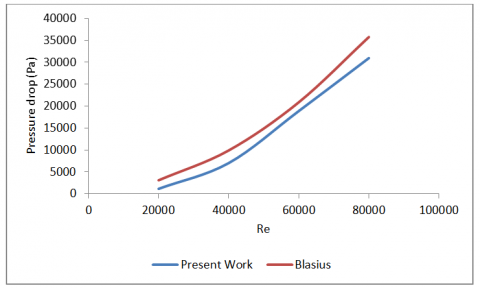Figure 14. Comparison of pressure drop with Blasius correlation for 2% vol

## 15.png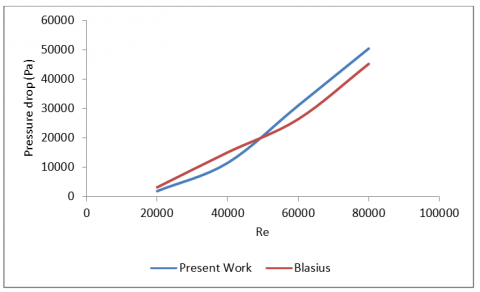Figure 15. Comparison of pressure drop with the Blasius correlation for 4% vol

## 16.png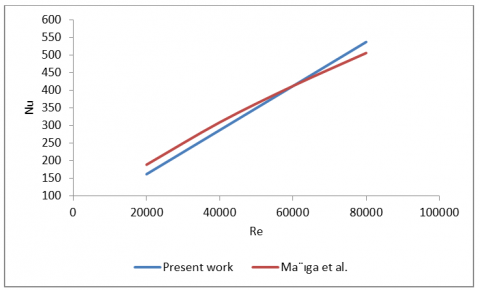Figure 16. Comparison of average Nu with the correlations for pure water

## 17.png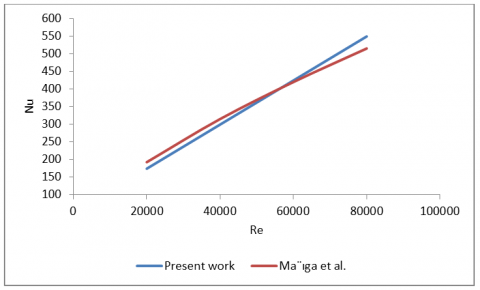Figure 17. Comparison of average Nu with the correlations for 2% vol

## 18.png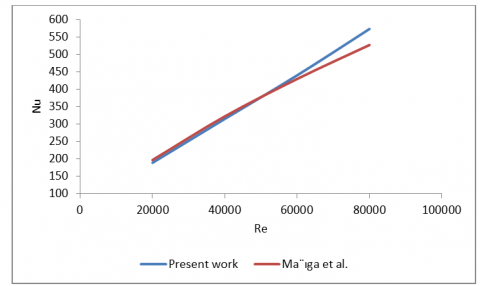Figure 18. Comparison of average Nu with the correlations for 4% vol

Figures 16, 17, and 18 show the Nu-Re results of 0, 2, and 4% vol., respectively with the experimental correlations proposed by Maïga et al. . The CFD results for the Nu show a maximum deviation of ±6.18, ±6.64, and ±8.74% for 0, 2, and 4% vol., respectively from the correlation.

4. Conclusions

Thermal analysis of heat transfer enhancement in subchannel geometry of pressurized water reactor using water-based Yttrium oxide nanofluid under steady-state turbulent flow condition, single phase, incompressible, and uniform heat flux. The CFD results are validated with the correlations in the literature and the conclusion can be listed as follows:

1. Using Yttrium oxide nanoparticles in the base fluid has an important duty in increasing the heat transfer rate. Although, the presence of nanoparticles in the coolant fluid increases the pressure drop if compared with the fresh fluid.
2. The increase in volume fraction of nanoparticles increases the heat transfer coefficient. As example, by increasing the volume fraction of nanoparticles from 0 to 2%, the heat transfer coefficient enhances by 20%.
3. The total pressure drop was raised gradually by increasing the volume fraction of nanoparticles. As example, by increasing the volume fraction of nanoparticles from 0 to 2%, 0 to 4% the total pressure drop increases by 19% and 94%, respectively.
4. The CFD results for the pressure drop show a maximum deviation of ±3.58, ±13.42, and ±11.56% for 0, 2, and 4% vol., respectively from the correlation. The CFD results for the Nu show a maximum deviation of ±6.18, ±6.64, and ±8.74% for 0, 2, and 4% vol., respectively from the correlation.
Acknowledgment

The authors would like to thank encouragement and assistance from their university, families, colleagues, friends, and tutors.

Nomenclature
 Cp Specific heat (J/kgK) D Diameter (m) Dh Equivalent hydraulic diameter (m) f Friction factor (….) g Acceleration of gravity (m/s2) h Heat transfer coefficient (W/m2 K) k Thermal conductivity (W/m K) Nu Nusselt number (….) P Mean pressure (Pa) Pr Prandtl number (….) Q Heat flux (W/m2) Re Reynolds number (….) T Temperature (K) ui Velocity vector (m/s) u´ Root-mean-square of the turbulent velocity fluctuations x, y, z Coordinates Greek Symbols μ Fluid dynamic viscosity (Pa·s) ρ Fluid density (kg/m3) $\varphi$ Volume fraction of nanoparticle Subscript av Averaged bf Base fluid b Bulk f Fluid nf Nanofluids p Particle w Wall 0 Initial value
References

 Jalili, P.S., Rahimi, S.A., Mohammadyari, R., Rahimi, E.M. (2015). Thermo-hydraulic investigation of nanofluid as a coolant in VVER-440 fuel rod bundle. Trans. Phenom. Nano Micro Scales, 3(2): 77-88. https://doi.org/10.7508/tpnms.2015.02.002

 Chen, Y.J., Li, Y.Y., Liu, Z.H. (2014). Numerical simulations of forced convection heat transfer and flow characteristics of nanofluids in small tubes using two-phase models. International Journal of Heat and Mass Transfer, 78: 993-1003. https://doi.org/10.1016/j.ijheatmasstransfer.2014.07.052

 Togun, H., Abu-Mulaweh, H.I., Kazi, S.N., Badarudin, A. (2016). Numerical simulation of heat transfer and separation Al2O3/nanofluid flow in concentric annular pipe. International Communications in Heat and Mass Transfer, 71: 108-117. https://doi.org/10.1016/j.icheatmasstransfer.2015.12.014

 Nayak, A.K., Kulkarni, P.P., Chinchole, A.S. (2015). Experimental investigation on pool boiling critical heat flux with nanofluids. Journal of Nanofluids, 4(2): 140-146. https://doi.org/10.1166/jon.2015.1145

 Vassallo, P., Kumar, R., D’Amico, S. (2004). Pool boiling heat transfer experiments in silica-water nano-fluids. International Journal of Heat and Mass Transfer, 47(2): 407-411. https://doi.org/10.1016/S0017-9310(03)00361-2

 You, S. M., Kim, J.H., Kim, K.H. (2003). Effect of nanoparticles on critical heat flux of water in pool boiling heat transfer. Applied Physics Letters, 83(16): 3374-3376. https://doi.org/10.1063/1.1619206

 Conner, M.E., Baglietto, E., Elmahdi, A.M. (2010). CFD methodology and validation for single-phase flow in PWR fuel assemblies. Nuclear Engineering and Design, 240(9): 2088-2095. https://doi.org/10.1016/j.nucengdes.2009.11.031

 Hadad, K., Kowsar, Z. (2015). Application of nanofluids as the primary coolant and neutron absorber in a PWR reactor. International Conference on Emerging Nuclear Energy Sciences, Istanbul-Turkey, pp. 1-9.

 Abdellahoum, C., Mataoui, A., Oztop, H.F. (2015). Turbulent forced convection of nanofluid over a heated shallow cavity in a duct. Powder Technology, 277: 126-134. https://doi.org/10.1016/j.powtec.2015.02.048

 Moghadassi, A., Ghomi, E., Parvizian, F. (2015). A numerical study of water based Al2O3 and Al2O3-Cu hybrid nanofluid effect on forced convective heat transfer. International Journal of Thermal Sciences, 92: 50-57. https://doi.org/10.1016/j.ijthermalsci.2015.01.025

 Zakir, M.G., Sarkar, M.R., Hossain, A. (2019). Analysis of Neutronics and Thermal-Hydraulic Behavior in a Fuel Pin of Pressurized Water Reactor (PWR). World Journal of Nuclear Science and Technology, 9(2): 74-83. https://doi.org/10.1016/10.4236/wjnst.2019.92005

 Sierchuła, J. (2019). Analysis of passive residual heat removal system in AP1000 nuclear power plant. In IOP Conference Series: Earth and Environmental Science, 214(1): 012095. https://doi.org/10.1088/1755-1315/214/1/012095

 Poudel, B., Joshi, K., Gokaraju, R. (2019). A Dynamic Model of Small Modular Reactor Based Nuclear Plant for Power System Studies. IEEE Transactions on Energy Conversion, 35(2): 977-985. https://doi.org/10.1109/TEC.2019.2956707

 Nazififard, M., Nematollahi, M., Suh, K.Y. (2011). Numerical analysis of water-based nanofluid coolant for small modular reactor. In Small Modular Reactors Symposium, 54730: 199-205. https://doi.org/10.1115/SMR2011-6662

 Maïga, S.E.B., Nguyen, C.T., Galanis, N., Roy, G., Maré, T., Coqueux, M. (2006). Heat transfer enhancement in turbulent tube flow using Al2O3 nanoparticle suspension. International Journal of Numerical Methods for Heat & Fluid Flow, 16(3): 275-292. https://doi.org/10.1108/09615530610649717

 Nazififard, M., Nematollahi, M., Jafarpur, K., Suh, K.Y. (2012). Computational analysis for research reactor IR-40 rod bundle. Atw. Internationale Zeitschrift fuer Kernenergie, 57(8-9): 523-529.

 Nematollahi, M.R., Nazifi, M. (2008). Enhancement of heat transfer in a typical pressurized water reactor by different mixing vanes on spacer grids. Energy Conversion and Management, 49(7): 1981-1988. https://doi.org/10.1016/j.enconman.2007.12.016

 Liu, C.C., Ferng, Y.M. (2010). Numerically simulating the thermal-hydraulic characteristics within the fuel rod bundle using CFD methodology. Nuclear Engineering and Design, 240(10): 3078-3086. https://doi.org/10.1016/j.nucengdes.2010.05.021

 Pak, B.C., Cho, Y.I. (1998). Hydrodynamic and heat transfer study of dispersed fluids with submicron metallic oxide particles. Experimental Heat Transfer an International Journal, 11(2): 151-170. https://doi.org/10.1080/08916159808946559

 Bianco, V., Chiacchio, F., Manca, O., Nardini, S. (2009). Numerical investigation of nanofluids forced convection in circular tubes. Applied Thermal Engineering, 29(17-18): 3632-3642. https://doi.org/10.1016/j.applthermaleng.2009.06.019

 Bianco, V., Manca, O., Nardini, S. (2011). Numerical investigation on nanofluids turbulent convection heat transfer inside a circular tube. International Journal of Thermal Sciences, 50(3): 341-349. https://doi.org/10.1016/j.ijthermalsci.2010.03.008

 Bianco, V., Manca, O., Nardini, S. (2010). Numerical simulation of water/Al2O3 nanofluid turbulent convection. Advances in Mechanical Engineering, 2: 976254. https://doi.org/10.1155/2010/976254

 Bianco, V., Nardini, S., Manca, O. (2011). Enhancement of heat transfer and entropy generation analysis of nanofluids turbulent convection flow in square section tubes. Nanoscale Research Letters, 6(1): 1-12. https://doi.org/10.1186/1556-276X-6-252

 Nazififard, M., Nematollahi, M., Jafarpour, K., Suh, K.Y. (2012). Augmented safety heat transport in research reactor IR-40 using nanofluid. Atw. Internationale Zeitschrift Fuer Kernenergie, 57(4): 262-270.